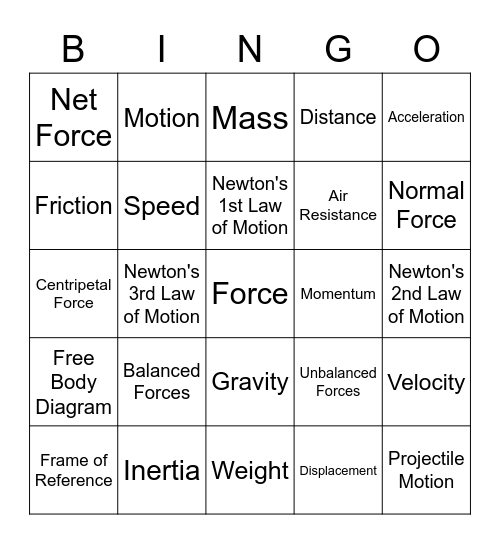# Force VocabularyThis bingo card has a free space and 24 words: Balanced Forces, Inertia, Newton's 1st Law of Motion, Frame of Reference, Acceleration, Net Force, Friction, Motion, Momentum, Gravity, Air Resistance, Centripetal Force, Unbalanced Forces, Velocity, Speed, Displacement, Newton's 2nd Law of Motion, Mass, Normal Force, Newton's 3rd Law of Motion, Projectile Motion, Free Body Diagram, Distance and Weight.

## Play Online